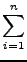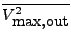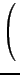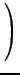Next: Weighting Functions Up: Definition of Dynamic Range Previous: Sinusoid with fixed frequency   Contents

### Noise

The filter output noise level is calculated from the input-referred noise spectra Sn, i of the intrinsic integrators and the transfer functions gi from the inputs of the intrinsic integrators to the output of the filter. If the noise spectra Sn, i are white, the output noise level of the filter is=Sn, i| gi|22. (24)
The dynamic range is

 DR =(25)
wherefollows either from (17) or from (21) or (23).

In the same way as | fi|22 is dependent on an input weighting function, | gi|22 is dependent on an output weighting function  So(ω) , which by default is identical to 1. Furthermore, one can prove  that

 Sn, i =| bi| +| aij|(26)
where Ci is the capacitance used for integrator i , and ξ is the noise factor.

FA will determine the dynamic range from the scaling flags, the total capacitance in the filter (the variable ctot), the maximal integrator output level (the variable umax) and the noise factor (the variable noisefactor) upon the command:

output: dr;
If the variable ctot is not set, it will generate an error message.Next: Weighting Functions Up: Definition of Dynamic Range Previous: Sinusoid with fixed frequency   Contents
2009-06-03